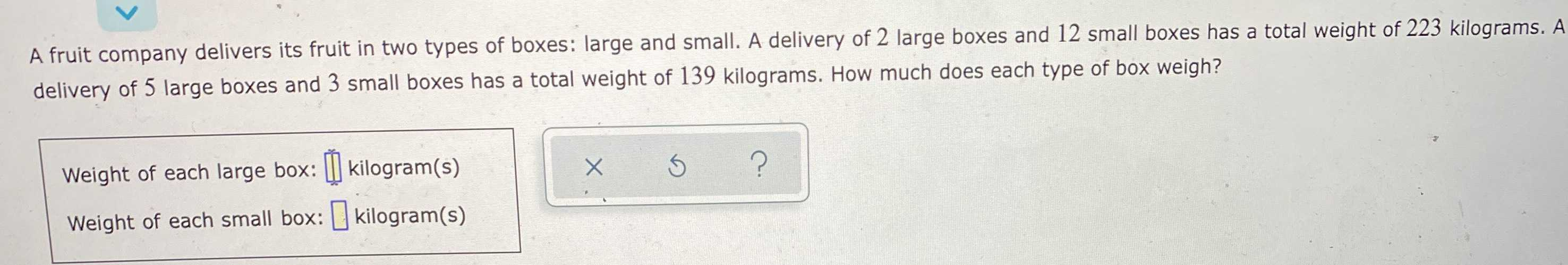### ¿Todavía tienes preguntas de matemáticas?

Pregunte a nuestros tutores expertos
Algebra
PreguntaA fruit company delivers its fruit in two types of boxes: large and small. A delivery of $$2$$ large boxes and $$12$$ small boxes has a total weight of $$223$$ kilograms. A delivery of $$5$$ large boxes and $$3$$ small boxes has a total weight of $$139$$ kilograms. How much does each type of box weigh?

Weight of each large box: $$\prod$$ kilogram(s)

Weight of each small box: $$\square$$ kilogram(s)